﻿ 基于Hilbert变换处理绝对重力仪测量数据文章快速检索 高级检索
 大地测量与地球动力学2019, Vol. 39Issue (6): 657-660  DOI: 10.14075/j.jgg.2019.06.021引用本文ZHENG Donglin, YANG Jinling, LI Song, et al. Absolute Gravimeter Measurement Data Processing Based on Hilbert-Transform[J]. Journal of Geodesy and Geodynamics, 2019, 39(6): 657-660.Corresponding author

YANG Jinling, associate professor, majors in laser remote sensing and photoelectric detection, E-mail:jlyang@whu.edu.cn.

About the first author

ZHENG Donglin, postgraduate, majors in laser ranging, E-mail: 361714806@qq.com.

文章历史

1. 武汉大学电子信息学院光电检测与激光遥感实验室，武汉市珞珈山，430079

1 算法原理 1.1 Hilbert变换原理

Hilbert变换定义为：

 $\begin{array}{l} \mathit{\tilde U}{\rm{(}}\mathit{t}{\rm{) =\mathit H}}\left\{ {\mathit{U}{\rm{(}}\mathit{t}{\rm{)}}} \right\} = h{\rm{(}}\mathit{t}{\rm{)}}\mathit{U}{\rm{(}}\mathit{t}{\rm{) = }}\\ \int_{ - \infty }^\infty U (\tau )h(t - \tau ){\rm{d}}\tau = \frac{1}{{\rm{ \mathit{ π} }}}\int_{ - \infty }^\infty {\frac{{U(\tau )}}{{t - \tau }}} {\rm{d}}\tau \end{array}$ (1)

 $H({\rm{j}}\omega ) = \left\{ \begin{array}{l} {\rm{j}}, \omega < 0\\ 0, \omega = 0\\ - {\rm{j}}, \omega > 0 \end{array} \right.$ (2)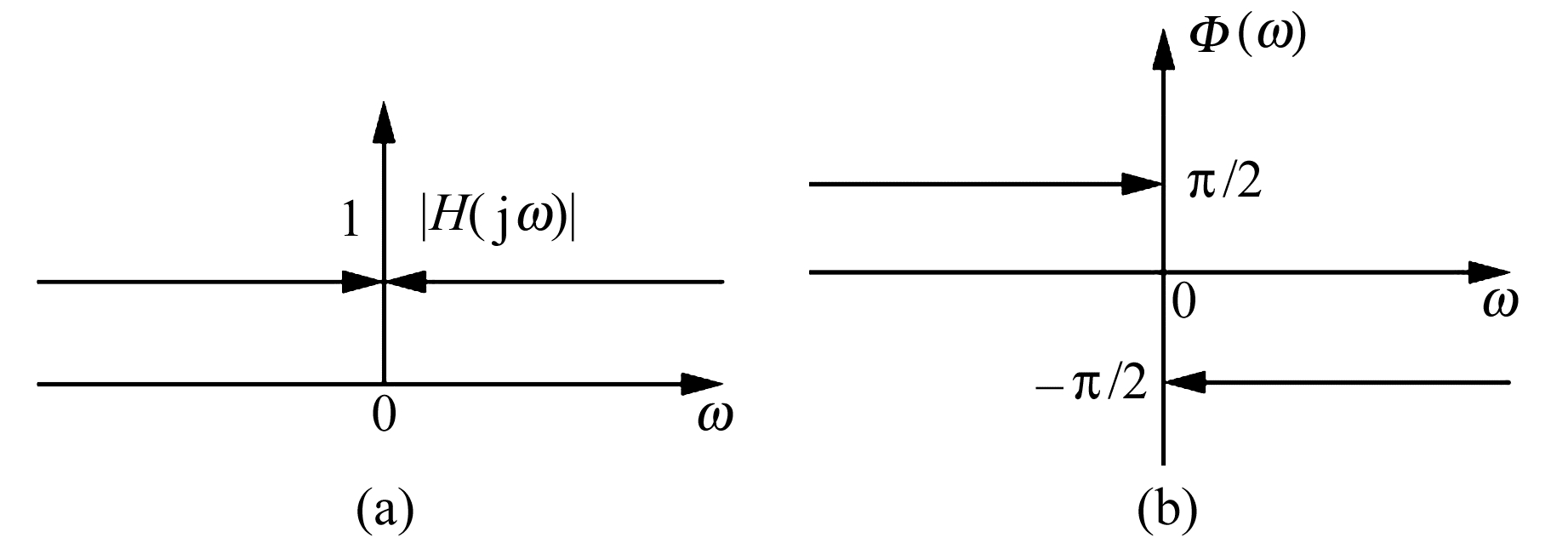图 1 Hilbert变换频率响应 Fig. 1 Frequency response of Hilbert-transform

 $\mathit{q}{\rm{(}}\mathit{t}{\rm{) = }}\mathit{U}{\rm{(}}\mathit{t}{\rm{) + i}}\mathit{\tilde U}{\rm{(}}\mathit{t}{\rm{)}}$ (3)

 $\mathit{\Phi }(t) = {\tan ^{ - 1}}(H\{ U(t)\} /U(t))$ (4)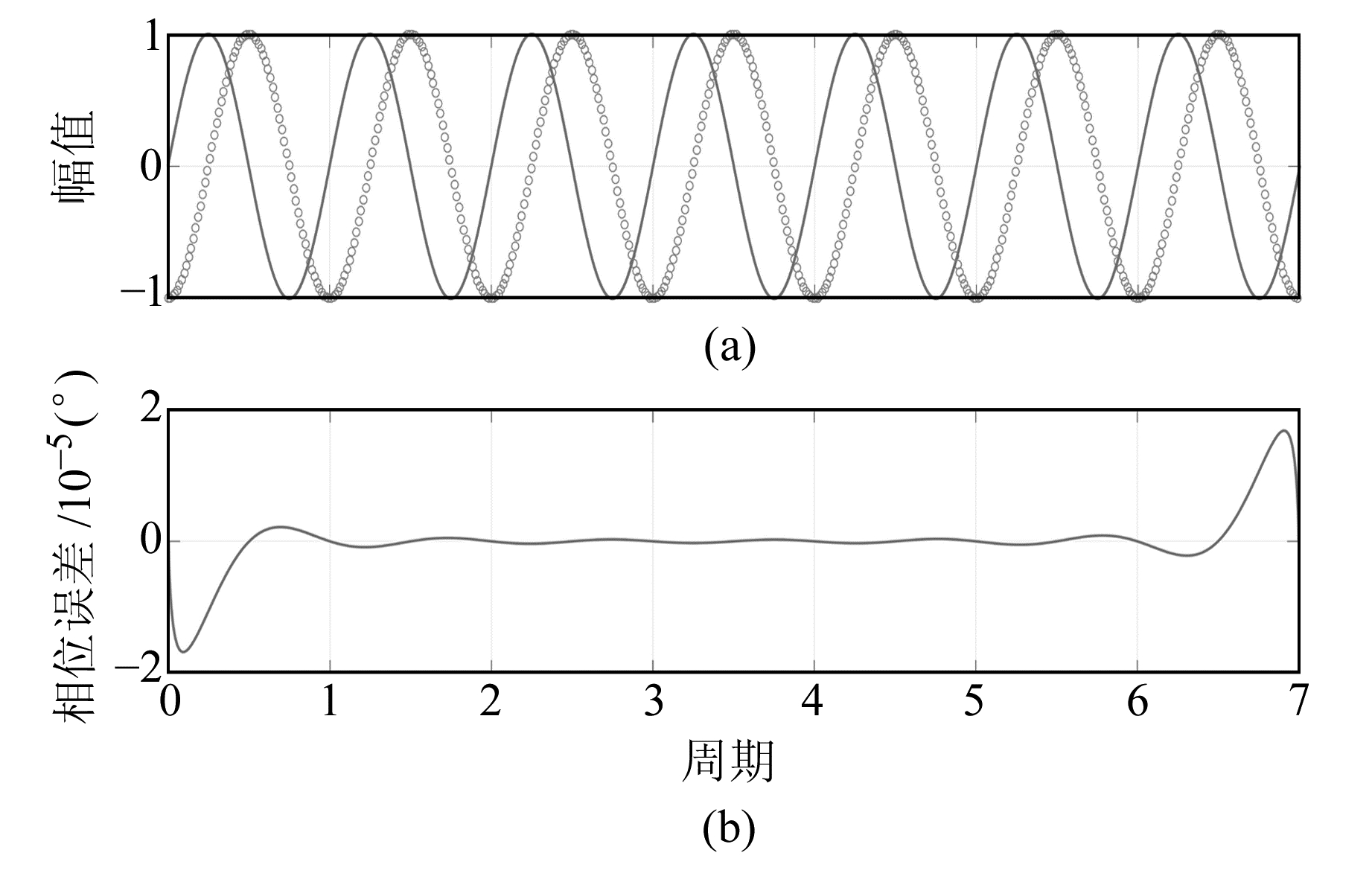图 2 正弦信号Hilbert变换的相移及误差 Fig. 2 Phase shift and error of sine signal's Hilbert-transfer
1.2 信号模型与时间修正

 $U(t) = {u_0} + \beta t + {A_0}\sin (\mathit{\Phi }(t))$ (5)

 $\mathit{\Phi }(t) = 2{\rm{ \mathit{ π} }}\frac{{S(t)}}{{\lambda /2}} = \frac{{4{\rm{ \mathit{ π} }}}}{\lambda }S(t)$ (6)

 $S(t) = \frac{{\lambda \mathit{\Phi }(t)}}{{4{\rm{ \mathit{ π} }}}}$ (7)

 ${S^\prime }(t) = \frac{{n\lambda }}{2} + S(t)$ (8)

 $S(t) = {s_0} + {v_0}t + \frac{1}{2}{g_0}{t^2}$ (9)

 $\begin{array}{l} {S^\prime }(t) = {s_0} + {v_0}t + \frac{1}{2}{g_0}{t^2} + \\ \gamma \left( {\frac{1}{2}{s_0}{t^2} + \frac{1}{6}{v_0}{t^3} + \frac{1}{{24}}{g_0}{t^4}} \right) \end{array}$ (10)

 $g = {g_0} + \gamma {h_{{\rm{ef}}}}$ (11)

 $t_i^\prime = t - \frac{{\Delta x}}{c} = t - \frac{{{s_i} - {s_0}}}{c}$ (12)

1.3 零相移滤波原理与去线性趋势处理

 ${x_1} = \left\{ {\begin{array}{*{20}{l}} {x\left( {n\% {n_0}} \right), n\% {n_0} \ne 0}\\ {x\left( {{n_0}} \right), n\% {n_0} = 0} \end{array}} \right.$ (13)

 $X(z) = \sum\limits_{n = - \infty }^\infty {{x_1}} (n){z^{ - n}}$ (14)
 $\begin{array}{l} Y(z) = \sum\limits_{n = - \infty }^\infty {{y_1}} (n){z^{ - n}} = \sum\limits_{n = - \infty }^\infty {{x_1}} ( - n){z^n} = \\ \;\;\;\;\;\;\;\;\sum\limits_{n = - \infty }^\infty {{x_1}} (n){\left( {{z^{ - 1}}} \right)^n} = X\left( {{z^{ - 1}}} \right) \end{array}$ (15)

 ${Y_1}(z) = X(z)H(z)H\left( {\frac{1}{z}} \right)$ (16)

 $\begin{array}{l} {Y_1}\left( {{{\rm{e}}^{{\rm{j}}\omega }}} \right) = X\left( {{{\rm{e}}^{{\rm{j}}\omega }}} \right)H\left( {{{\rm{e}}^{{\rm{j}}\omega }}} \right)H\left( {{{\rm{e}}^{ - {\rm{j}}\omega }}} \right) = \\ \;\;\;\;\;\;\;\;\;\;X\left( {{{\rm{e}}^{{\rm{j}}\omega }}} \right){\left| {H\left( {{{\rm{e}}^{{\rm{j}}\omega }}} \right)} \right|^2} \end{array}$ (17)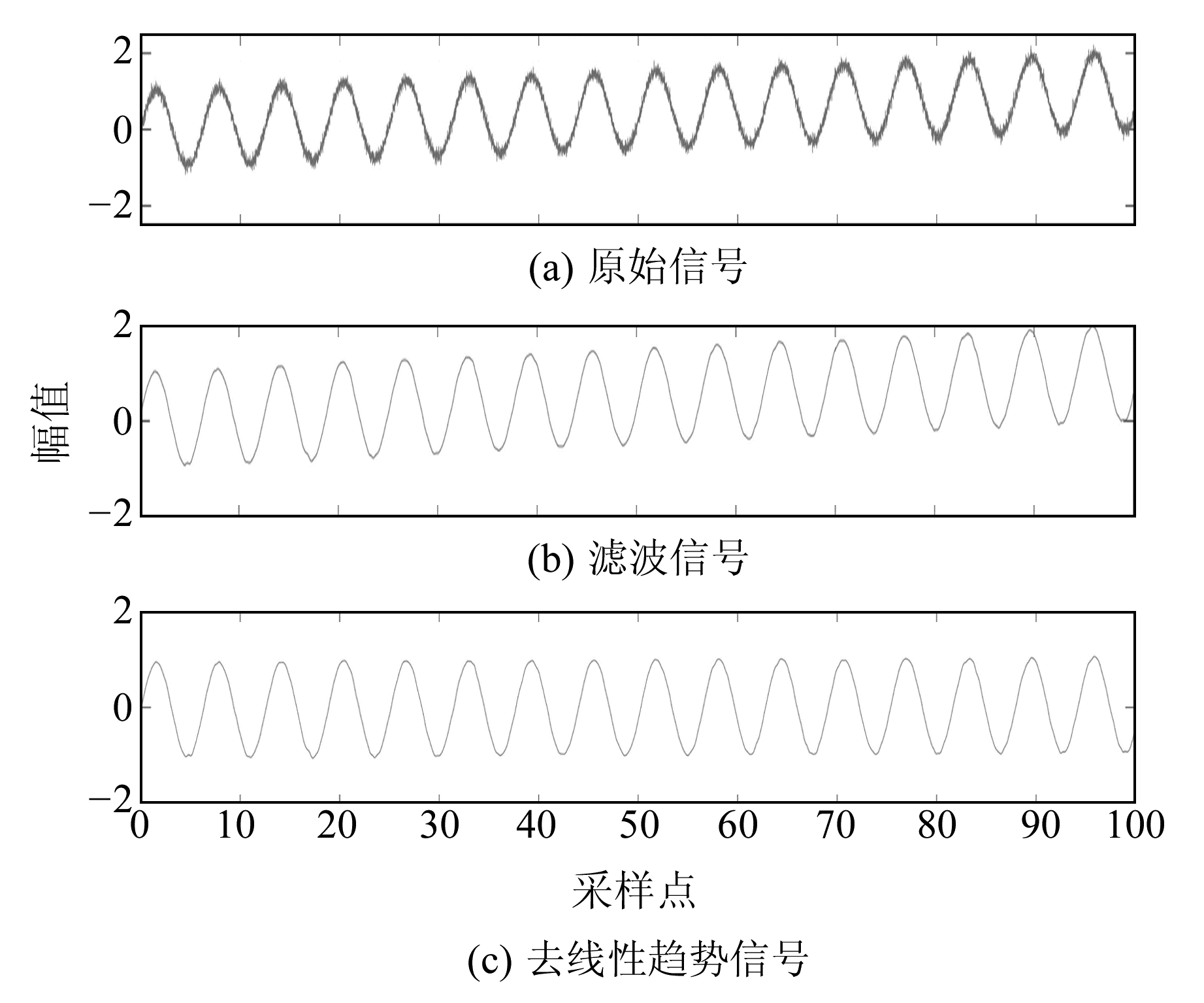图 3 信号高频噪声滤波以及线性噪声去除 Fig. 3 High frequency noise of signal filtering and linear trend removal
1.4 数据处理流程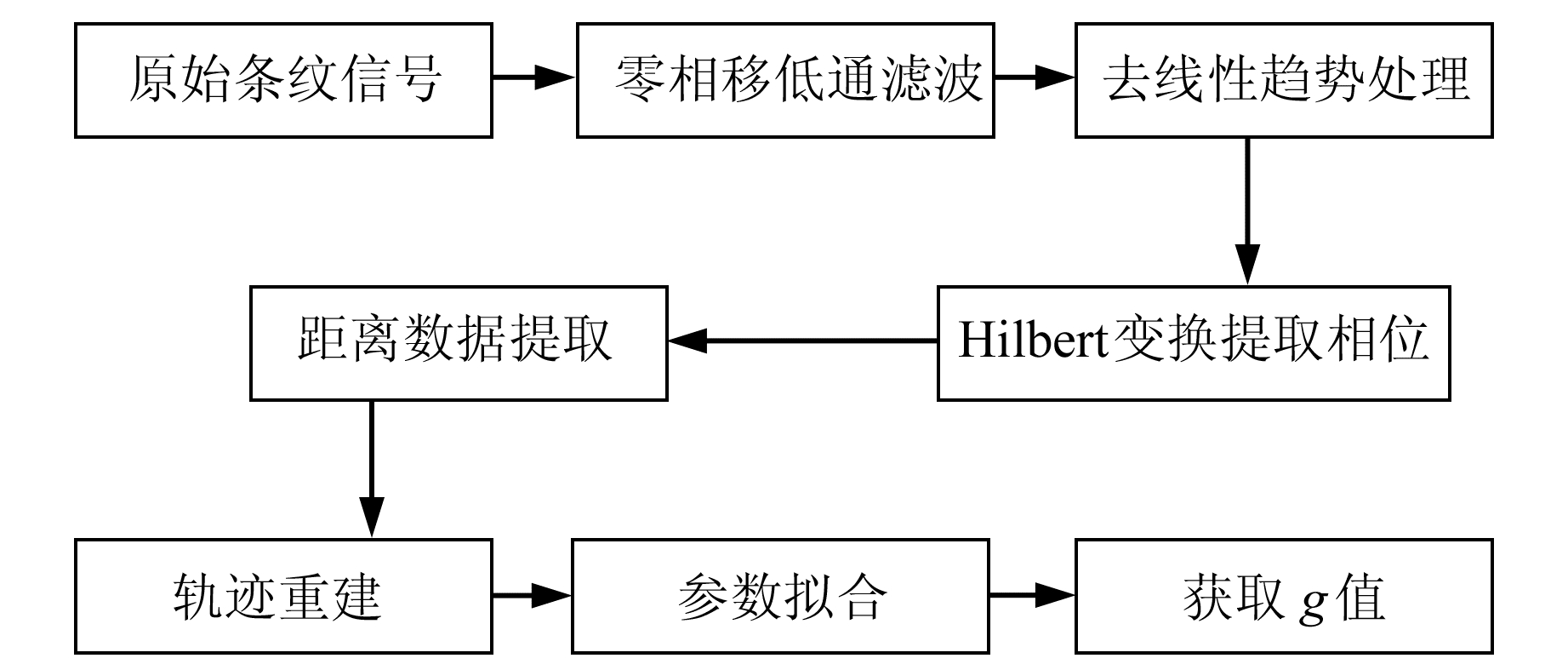图 4 数据处理流程 Fig. 4 The flow chart of data processing
2 仿真与实验结果分析 2.1 仿真结果与分析表 1 g值仿真结果 Tab. 1 Simulation results of g values

 ${\sigma _g} = \frac{{12\sqrt 5 }}{{{T^2}}} \cdot \frac{{{\sigma _S}}}{{\sqrt N }}$ (18)

2.2 实验结果与分析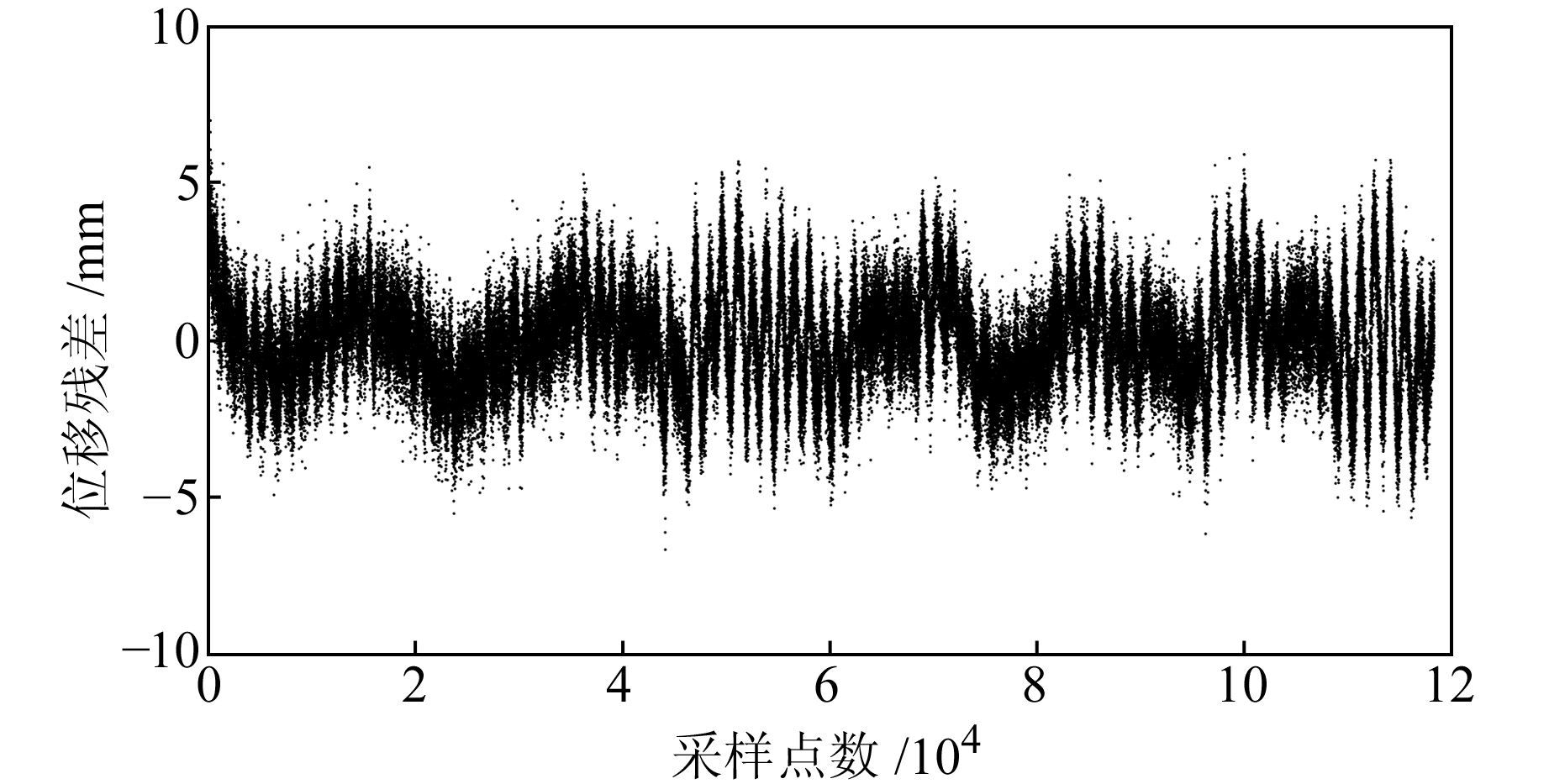图 5 最小二乘拟合位移残差 Fig. 5 Residual of the least squares fitting displacement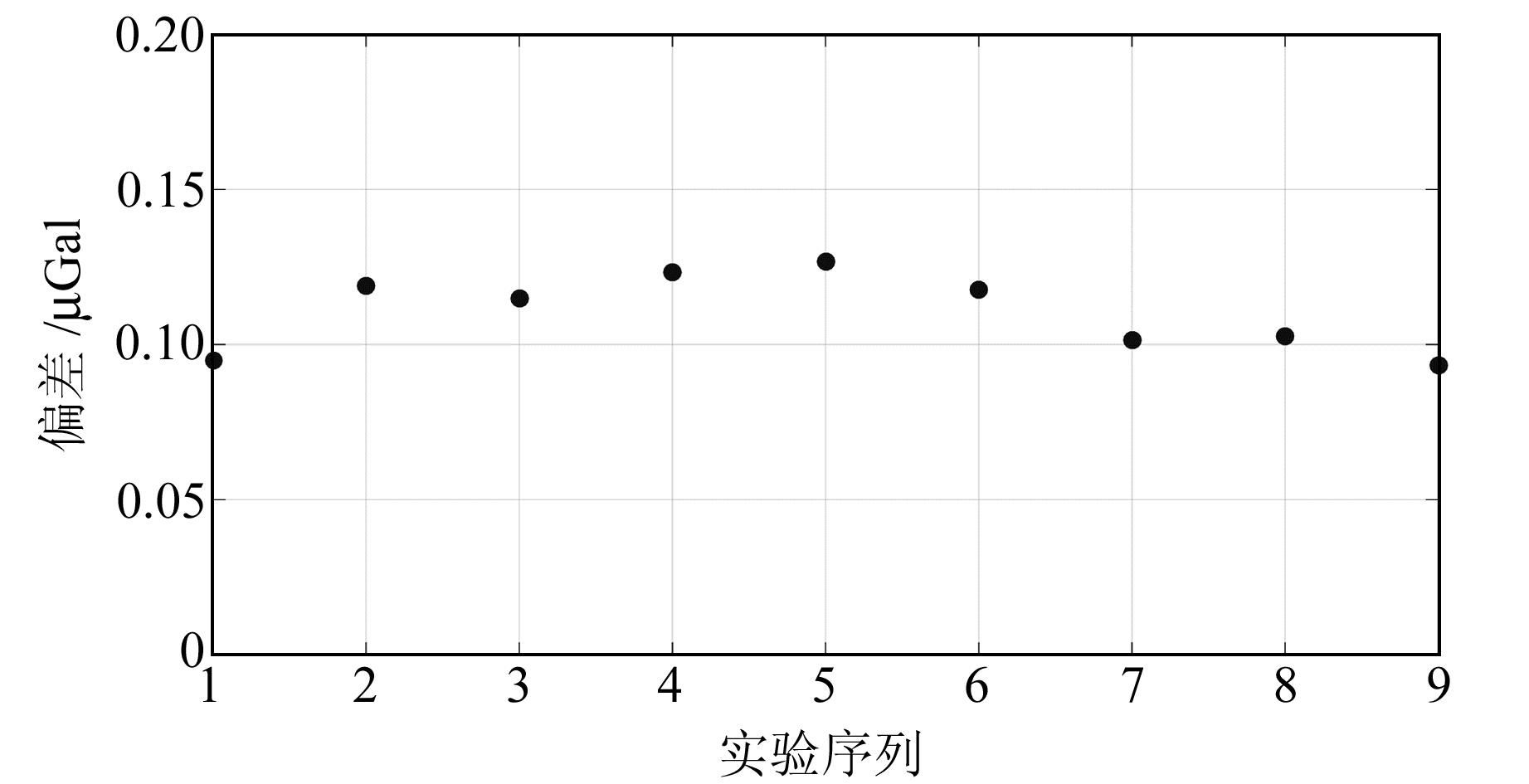图 6 实验得到的g值偏差σg Fig. 6 σg of g values obtained from the experiment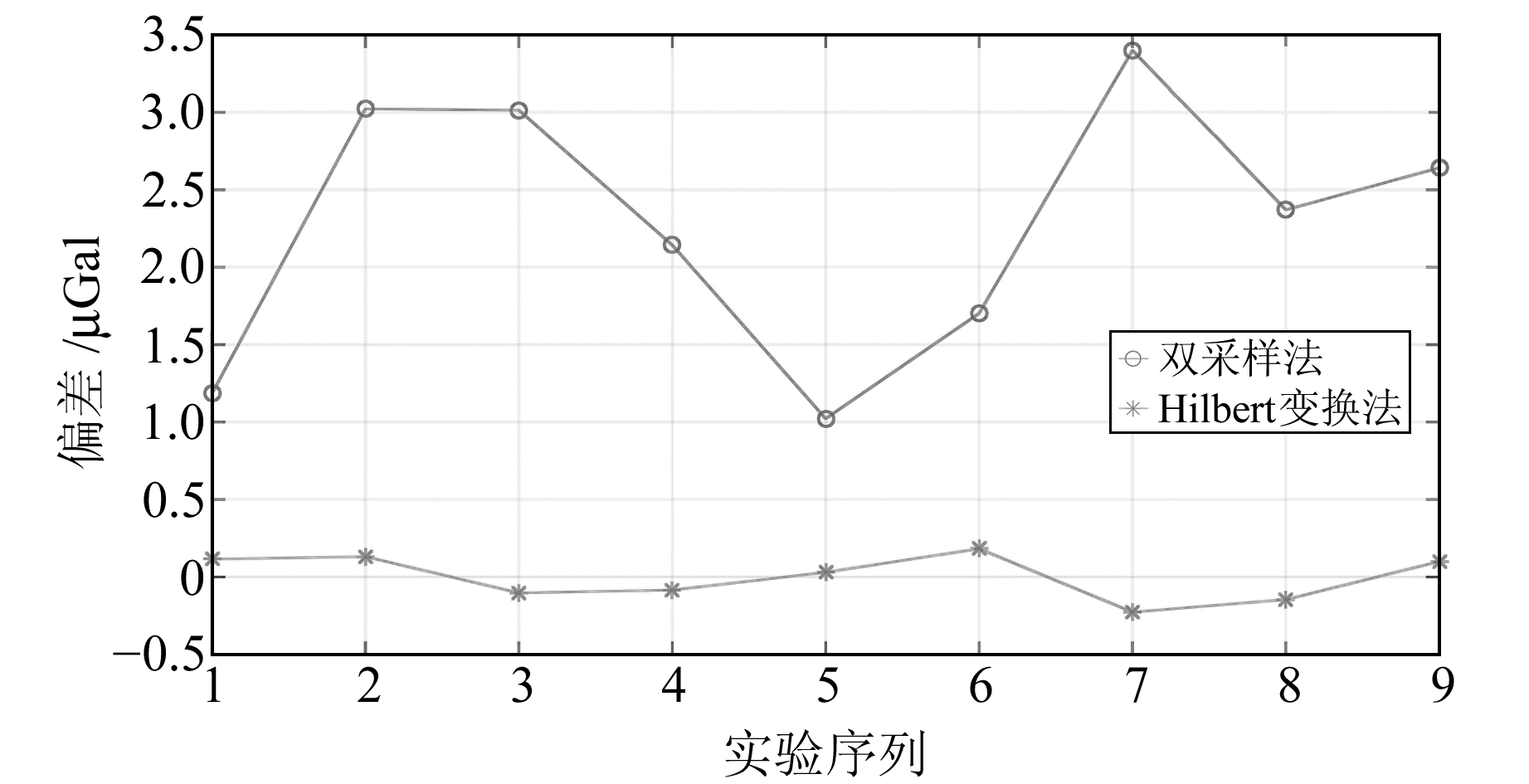图 7 双采样法与Hilbert变换法结果误差 Fig. 7 Errors of two-sample method and Hibert-transfer method
3 结语

  Svitlov S, Masłyk P, Rothleitner C, et al. Comparison of Three Digital Fringe Signal Processing Methods in a Ballistic Free-Fall Absolute Gravimeter[J]. Metrologia, 2011, 47(6): 677 (0)  Svitlov S, Araya A. Homodyne Interferometry with Quadrature Fringe Detection for Absolute Gravimeter[J]. Applied Optics, 2014, 53(16): 3 548-3 555 DOI:10.1364/AO.53.003548 (0)  Schoukens J, Renneboog J. Modeling the Noise Influence on the Fourier Coefficients after a Discrete Fourier Transform[J]. IEEE Transactions on Instrumentation & Measurement, 1986, 35(3): 278-286 (0)  Murata I. A Transportable Apparatus for Absolute Measurement of Gravity[J]. Bulletin of the Earthquake Research Institute University of Tokyo, 1978, 53(1): 49-130 (0)  罗志才, 宛家宽. 自由落体式绝对重力仪测量数据处理方法研究[J]. 大地测量与地球动力学, 2016, 36(3): 189-192 (Luo Zhicai, Wan Jiakuan. Research on Free-Fall Absolute Gravimeter Data Processing Method[J]. Journal of Geodesy and Geodynamics, 2016, 36(3): 189-192) (0)  Svitlov S, Rothleitner C, Wang L J. Accuracy Assessment of the Two-Sample Zero-Crossing Detection in a Sinusoidal Signal[J]. Metrologia, 2012, 49(4): 413-424 DOI:10.1088/0026-1394/49/4/413 (0)  Svitlov S, Araya A, Tsubokawa T. Digital Fringe Signal Processing Methods in Absolute Gravimetry[C]. IAG Symposium on Terrestrial Gravimetry: Static and Mobile Measurements (TG-SMM 2013), Saint Petersbourg, 2013 (0)
Absolute Gravimeter Measurement Data Processing Based on Hilbert-Transform
ZHENG Donglin1YANG Jinling1LI Song1     ZHANG Wenhao1
1. Photoelectric Testing and Laser Remote Sensing Laboratory, School of Electronic Information, Wuhan University, Luojia Hll, Wuhan 430079, China
Abstract: The instantaneous phase of interference fringe signal sampling point is extracted based on the phase shift principle of Hilbert-transfer, the displacement data is extracted from the phase, and time data is extracted from the sample frequency. We rebuild the free-falling trajectory by means of the least square method, so that the value of g can be calculated. Zero phase-shift low-pass filter is used to remove high frequency noise signal, the polynomial fitting method is used to remove linear interference signal, and data is windowed before fitting to inhibit the Gibbs-phenomenon. The simulation results show that this method can make the g value error under 10-2 μGal. The experimental results obtained mean error of the g value is 0.12 μGal.
Key words: absolute gravimeter; digital interference fringe; Hilbert-transform; data windowed